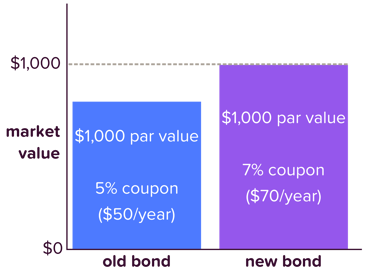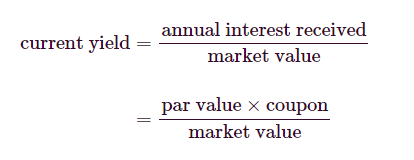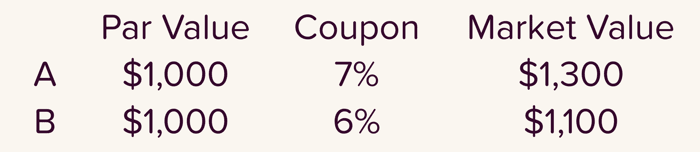-->

# BOND VALUES AND YIELDS

10:31

## Introduction: Bond Values and Yields

In our previous post, we discussed what bonds are and how they are different from stocks. In this lesson, we will be discussing how bonds are valued from which you will understand the relationship between interest rates, bond values and yields. You will also learn how to calculate and interpret current yield and yield to maturity. Let's dive in!Bond | Photo from Adobe Stock

We will be using Uche has our hypothetical investor

### Type of Bond Markets (Primary and Secondary)

Uche (our investor) can buy and sell bonds in much the same way that he trades stocks. He can purchase bonds directly from the issuing entity on the primary market or trade them with other investors on the secondary market

### Bond Issuance and Par Value (Face Value of Bonds)

Corporate bonds are issued with par values of \$1,000. par value remains constant throughout the life of a bond. The par or face value is written on the face of the bond—at least it was when bonds were made out of paper. The par value cannot change.

Like stocks, a bond's market value—the price willing buyers and sellers will trade at—will fluctuate away from its par value almost immediately after the bond is issued. Bonds are bought and sold at market value, which may or may not equal par value. If the market value is above the par value, the bond is traded at a premium. If the market value is below the par value, the bond is traded at a discount. Regardless of the purchase price, a bondholder receives the par value at maturity.

### Bond's Coupon, Market Value and Interest Rate

Like its par value, a bond's coupon also remains constant. A bond with a \$1,000 par value and 5% coupon always pays out \$50 in interest every year. A bond's changing market value, then, is not based on par value and coupon alone. Rather, one of the biggest drivers of market value is how interest rates in the broader economy are changing. When issued, a bond's coupon is usually set to match the interest rate of similar investments so that the initial market value of the bond will equal the par value of \$1,000. Bonds issued at different times will, therefore, have different coupons corresponding to the market rate when they're issued.Market of bond

Suppose a previously-issued bond has a 5% coupon. Uche (the investor) is considering purchasing this bond when interest rates in the broader economy have risen to 7%. In other words, bonds currently being issued have 7% coupons. Should Uche pay par value for the 5% bond when that same \$1,000 can purchase other bonds with 7% coupons? The answer is "NO" because why would any investor spend \$1,000 for a \$50 annual payment when \$70 payments are available for the same investment? Uche should only buy the 5% bond at a discount.

Likewise, Uche is willing to pay a premium for a bond offering a 5% coupon in a market with interest rates of only 3%. A \$50 annual payment is worth more than a \$30 annual payment. Because a bond's market value equals the price buyers are willing to pay and sellers are willing to take, we can conclude that the market values of bonds that have already been issued:

• Increases as interest rates decreases —a 5% bond becomes very attractive to investors when interests rates drop to 3%.
• Decreases as interest rates increases—no one wants to pay par value for a 5% bond when they could buy a 7% bond at par value instead.

### How to Calculate Bond's Current Yield

When the market value of a bond—that is, its purchase price—does not equal par value, a bond's coupon will not tell investor Uche the annual rate of return he can expect from purchasing a bond. A 5% coupon means a bondholder gets 5% of the par value in interest every year. If the bond isn't purchased at par value, the interest payments will not equal 5% of the purchase price.

So, what Uche wants is the current yield. While the coupon expresses a bond's annual interest payment as a percentage of  par value, current yield expresses it as a percentage of market value.

Current yield is calculated by dividing a bond's annual interest payments by its market value which, as we've seen, is most likely not equal to its par value.Bond current yield

A bond with a par value of \$1,000 and a 3% coupon that has a market value of \$900 has a current yield of [(\$1,000 * 3%) / \$900] = \$30 / \$900 = 3.33%

Suppose the market value of the bond fell from \$900 to \$600. It still pays \$30 in interest every year—the coupon doesn't change, remember—but now the bond's current yield is \$30 / \$600 = 5%Comparing Bond Current Yield

Uche is deciding between Bond A and Bond B. Their vitals are listed above.
• A has a current yield of \$70 / \$1,300 = 5.38%
• B has a current yield of \$60 / \$1,100 = 5.45%
If Uche is only concerned with the return he'll receive in the next year, Bond B is his best bet. He'll make a greater return over the year by investing in Bond B at these market values.

### How  to Calculate Bond's Yield to Maturity (YTM)

Current yield is handy if Uche is only looking at the next year of returns. If he wants to go beyond that, he turns to the yield to maturity (YTM). Yield to maturity calculates the total return an investor will receive from a bond he holds until maturity, taking into account all future interest payments as well as the bond's par and market values. Notice the difference in the time element between current yield and yield to maturity.

### Difference Between Current Yield and Yield to Maturity

While current yield assumes Uche holds a bond for a year, yield to maturity assumes that Uche holds a bond until maturity. Despite its long-term aspect, YTM is expressed as an annual rate.

The yield to maturity calculation is complicated, and Uche lets his computer handle it. What he wants us to take away is that YTM assumes that Uche will reinvest all of his interest payments at the same rate as the current yield. If Uche uses his interest payments to fund his ever-growing cufflink and pocket square collection, yield to maturity won't be accurate.

Whenever the market value of a bond equals its par value, both current yield and yield to maturity equal the bond's coupon. Like the coupon, both yields are expressed as annual returns. Since there is no change in the bond's price, the coupon remains the annual return rate.
We know that changing interest rates in the broader economy affect a bond's market value. Therefore, changing interest rates also affect yields.

Uche is considering purchasing a bond with a \$1,000 par value, a 6% coupon, and a maturity date in 4 years. Interest rates have risen since the bond was issued and the bond is currently valued at \$800.
The bond's current yield is \$60 / \$800 = 7.5%
(we divide the annual return in the current yield calculation. This bond pays 6% of \$1,000, or \$60 and divide the annual payments by the current market value.)
The Yield to Maturity is 12.68% ( remember that YTM includes all future interest payments, reinvesting those payments, and the fact that C.B. will receive the full par value at maturity—\$200 over the purchase price.)

### How Changes in Interest Rate Affects Bond's Market Value and Yield

Interesting! A rise in interest rates caused the bond's market value to drop from \$1,000 to \$800, but led its current yield to rise  from 6% to 7.5%. Its yield to maturely rose from 6% to 12.68%.

A fall in interest rates in the broader economy lead will to:

• A rise in bond market values. —Bond values move in the opposite direction as interest rates in the broader economy.
• A fall in bond yields.  —Bond yields follow the interest rates in the broader economy.

Let's make sure we've got all this down. The following statements are classified as either true or false.

TRUE FALSE
The current yield is the return an investor can expect by buying a bond and holding it for one year. A bond purchased at a discount is purchased above par value.  (A bond purchased above par value is made at a premium and a purchase below par value is made at a discount)
If a bond is purchased at par value, current yield and yield to maturity will equal the bond's coupon. The yield to maturity is the return an investor can expect by buying a bond, holding it until maturity, and spending the interest payments. (The interest payments need to be reinvested at the current yield for the yield to maturity calculation to work.)
As interest rates in the broader economy rise, current yield and yield to maturity also rise. As interest rates in the broader economy fall, a bond's market value will also fall. (Market values and interest rates move in opposite directions.)

In the next lesson, we'll meet a few other types of bonds hanging out in the market. Are you ready? Let's go!